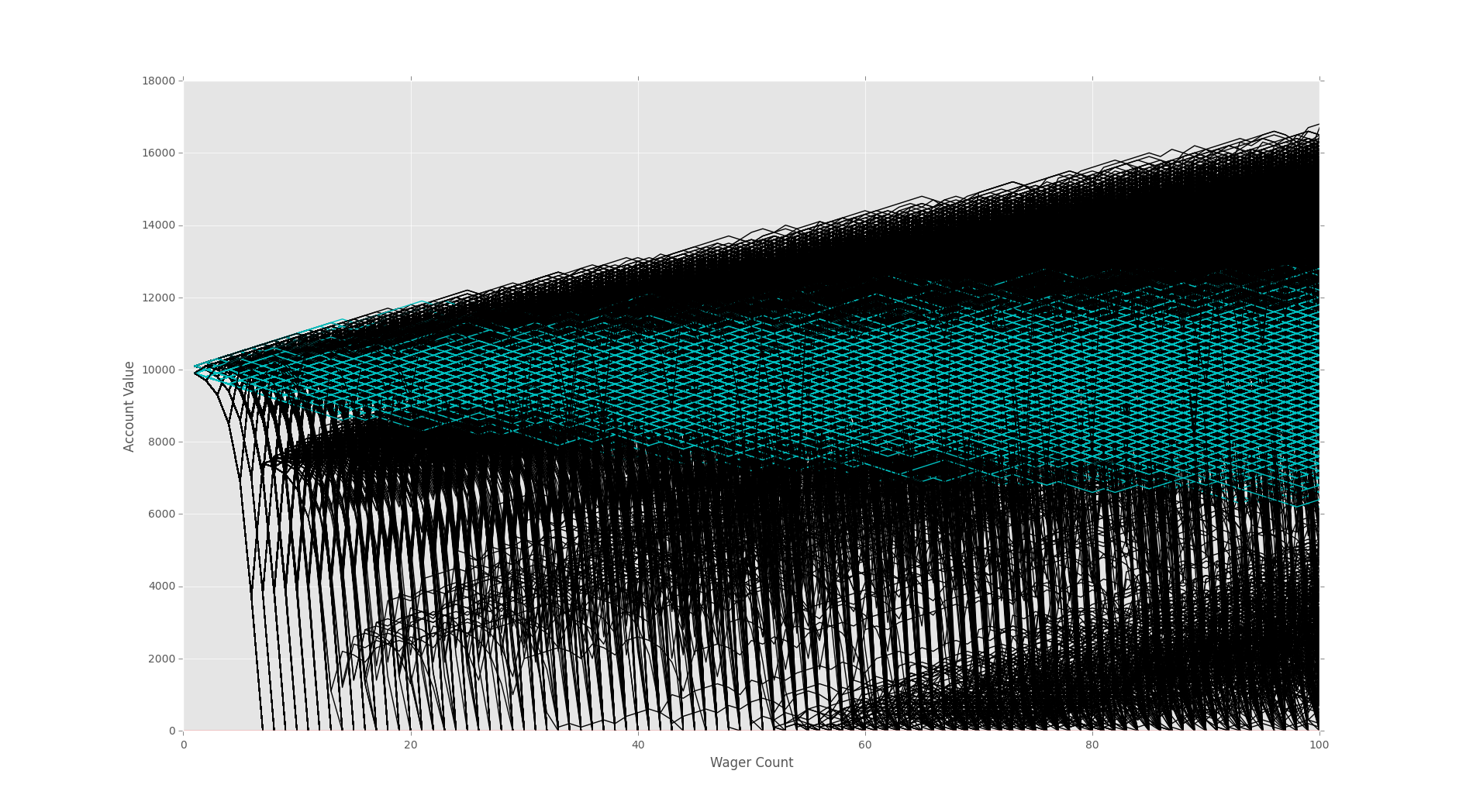## Analyzing Monte Carlo results

So now that we've compared the life expectency of these strategies, we should also compare profits. First we can just compare profit vs loss, but also degree of profits is important. Both strategies stand to lose equal amounts, but this is not the case for gains. It is clear that our doublor bettor, despite having lower life expectancy, has far more upside compared to the simple bettor.

```import random
import matplotlib
import matplotlib.pyplot as plt
import time

sampleSize = 10000
startingFunds = 10000
wagerSize = 100
wagerCount = 100

def rollDice():
roll = random.randint(1,100)

if roll == 100:
return False
elif roll <= 50:
return False
elif 100 > roll >= 50:
return True

def doubler_bettor(funds,initial_wager,wager_count,color):
global doubler_busts
#####################
global doubler_profits
value = funds
wager = initial_wager
wX = []
vY = []
currentWager = 1
previousWager = 'win'
previousWagerAmount = initial_wager

while currentWager <= wager_count:
if previousWager == 'win':
if rollDice():
value += wager
wX.append(currentWager)
vY.append(value)
else:
value -= wager
previousWager = 'loss'
previousWagerAmount = wager
wX.append(currentWager)
vY.append(value)
if value < 0:
currentWager += 10000000000000000
doubler_busts += 1
elif previousWager == 'loss':
if rollDice():
wager = previousWagerAmount * 2
if (value - wager) < 0:
wager = value

value += wager
wager = initial_wager
previousWager = 'win'
wX.append(currentWager)
vY.append(value)
else:
wager = previousWagerAmount * 2
if (value - wager) < 0:
wager = value
value -= wager
previousWager = 'loss'
previousWagerAmount = wager
wX.append(currentWager)
vY.append(value)

if value <= 0:
currentWager += 10000000000000000
doubler_busts += 1

currentWager += 1
plt.plot(wX,vY,color)
#####################
if value > funds:
doubler_profits+=1

'''
Simple bettor, betting the same amount each time.
'''

def simple_bettor(funds,initial_wager,wager_count,color):
global simple_busts
#####################
global simple_profits

value = funds
wager = initial_wager
wX = []
vY = []
currentWager = 1
while currentWager <= wager_count:
if rollDice():
value += wager
wX.append(currentWager)
vY.append(value)
else:
value -= wager
wX.append(currentWager)
vY.append(value)

if value <= 0:
currentWager += 10000000000000000
simple_busts +=1
currentWager += 1
plt.plot(wX,vY,color)
#####################
if value > funds:
simple_profits+=1

x = 0

simple_busts = 0.0
doubler_busts = 0.0

#####################
simple_profits = 0.0
doubler_profits = 0.0

while x < sampleSize:
simple_bettor(startingFunds,wagerSize,wagerCount,'c')
#simple_bettor(startingFunds,wagerSize*2,wagerCount,'c')
doubler_bettor(startingFunds,wagerSize,wagerCount,'k')
x+=1

print(('Simple Bettor Bust Chances:', (simple_busts/sampleSize)*100.00))
print(('Doubler Bettor Bust Chances:', (doubler_busts/sampleSize)*100.00))

print (('Simple Bettor Profit Chances:', (simple_profits/sampleSize)*100.00))
print(('Doubler Bettor Profit Chances:', (doubler_profits/sampleSize)*100.00))

plt.axhline(0, color = 'r')
plt.ylabel('Account Value')
plt.xlabel('Wager Count')
plt.show()
```

Your results may vary slightly, but they should be something like:

```('Simple Bettor Bust Chances:', 0.0)
('Doubler Bettor Bust Chances:', 31.269999999999996)
('Simple Bettor Profit Chances:', 38.32)
('Doubler Bettor Profit Chances:', 63.190000000000005)
```The next tutorial:• Monte Carlo Introduction

• Monte Carlo dice Function

• Creating a simple Bettor

• Plotting Results with Matpltolib

• Martingale Strategy

• Bettor Statistics

• More comparison

• Graphing Monte Carlo

• Fixing Debt Issues

• Analyzing Monte Carlo results
• Using Monte Carlo to find Best multiple

• Checking betting results

• D'Alembert Strategy

• 50/50 Odds

• Analysis of D'Alembert

• Comparing Profitability

• Finding best D'Alembert Multiple

• Two dimensional charting monte carlo

• Monte Carlo Simulation and Python

• Labouchere System for Gambling Tested